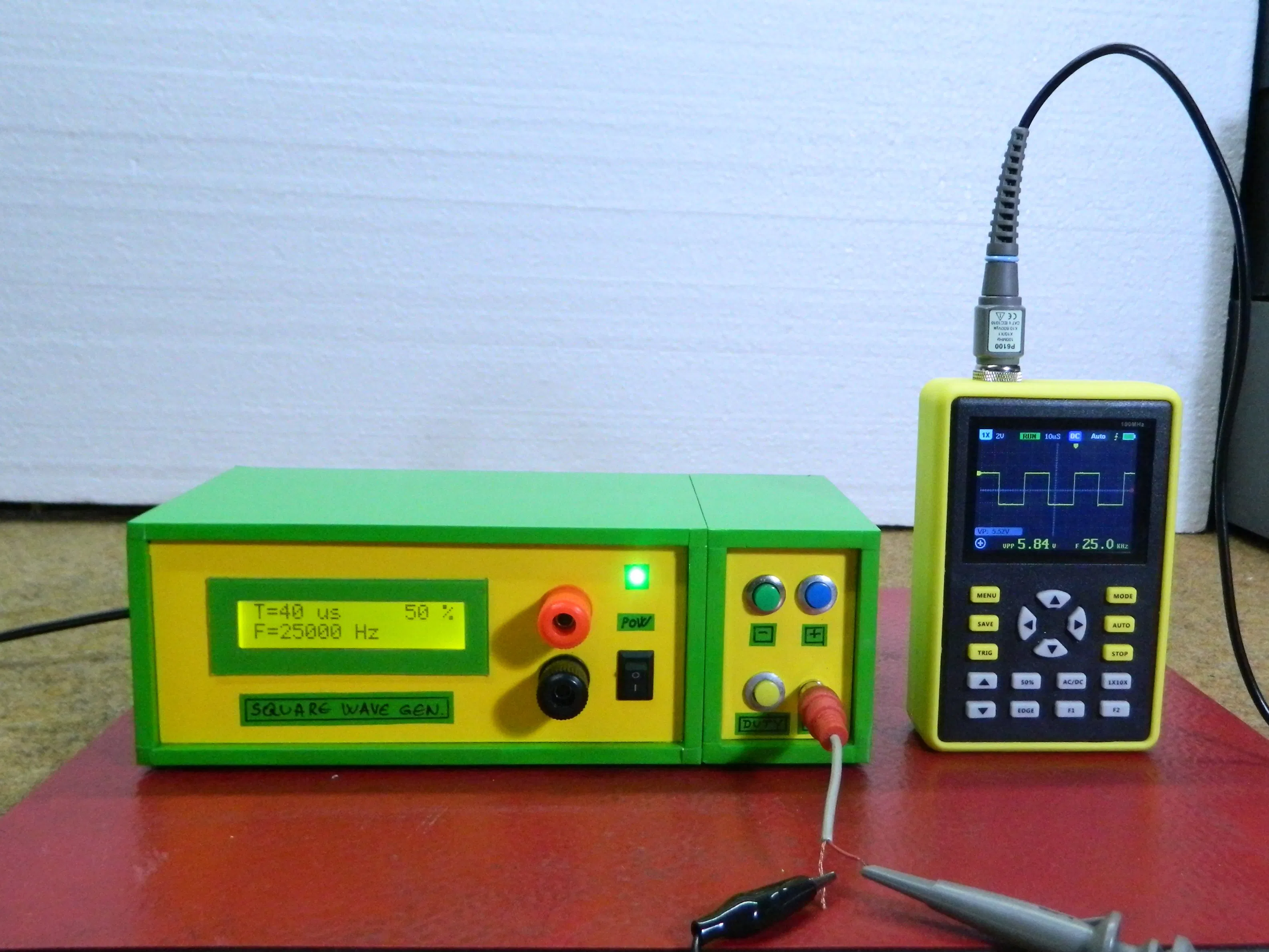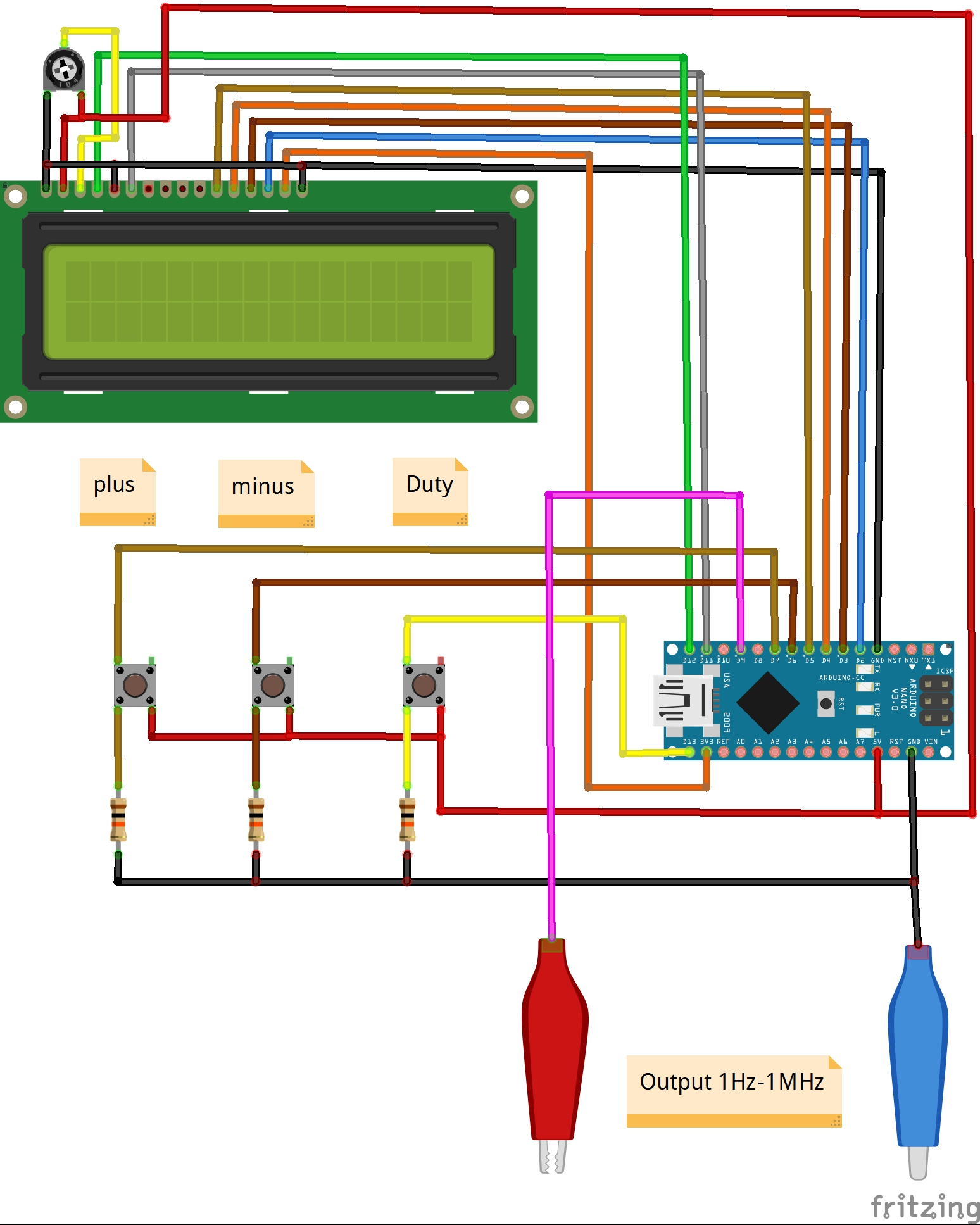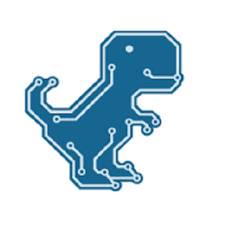Project tutorial# DIY Simple Square Wave Generator Up to 1MHz © GPL3+

This is a simple square-wave generator that basically uses the TimerOne library allow you to generate a PWM signal at pin 9.

• 5,003 views
• 18 respects

## Apps and online services

This is a simple square-wave generator that basically uses the TimerOne library allow you to generate a PWM signal at pin 9 in the range from about 5Hz to 1 Mhz, and you can adjust the duty cycle from 0 to 100%.

Device is very simple to build and consist only a few components:

- Arduino Nano microcontroller

- LCD display

- Three pull up resistors

- and three push buttons

The pulse generator has the ability to adjust the pulse repetition period using the buttons connected to digital inputs 6 and 7 of the Arduino. 13 input pin allows you to adjust the duty cycle. The duration and duty cycle readings are displayed on the first row of the LCD 16 × 2 indicator, and the frequency readings are displayed in the second row. The minimum step for adjusting the pulse repetition period is 1 μs, so the frequency will change discretely, for example, 1 μs is 1 MHz, 2 μs is 500 kHz, 3 μs is 333.333 Hz, and so on, and as the frequency decreases, the smoothness of its adjustment increases. This is quite impractical at higher frequencies but that is the price of simplicity. In one of my previous videos I have described the construction of a similar device but with the help of a specialized DDS chip that does not have these shortcomings and has a much larger range, but is more complex to build

To visualize the output signal I use small single- channel oscilloscope. Finally, the device is mounted in a suitable box, and it is another useful tool in the electronics laboratory.

## Code

##### CodeC/C++
```#include <TimerOne.h>
#include <LiquidCrystal.h>
LiquidCrystal lcd(12, 11, 5, 4, 3, 2);// RS,E,D4,D5,D6,D7
unsigned long t=1000,f,k=512;// default 1000 μs (1000 Hz), meander, pulse

duration is equal to duty cycle k = 512 (50%)
byte k1,kn,kn1,kn2;
int drive,drive0;

void setup()
{
lcd.begin(16, 2);// LCD 16X2
pinMode(9, OUTPUT);
pinMode(6,INPUT);// button at input 6
pinMode(7,INPUT);// button at input 7
pinMode(13,INPUT);// button at input 13
}
void loop()
{
Timer1.initialize(t); // period
Timer1.pwm(9, k); // k - fill factor 0-1023.
kn=digitalRead(6);// button input 6 (- pulse period)
kn1=digitalRead(7);// button input 7 (+ pulse period)
kn2=digitalRead(13);// button input 13 (+ circle fill factor)

if(kn==HIGH){ // decreasing the period
drive++;
if(drive<30){
t=t-1;
}
// if the button is held for a long time, the correction of the pulse

period x10 x100 x1000 is accelerated
else if(drive>30 && drive<60 ){
t=t-10;
}
else if(drive>=60 && drive<100){
t=t-100;
}
else if(drive>=100){
t=t-1000;
}
}
else{
drive=0;
}

drive0++;
if(drive0<30){
t=t+1;
// if the button is held for a long time, the correction of the

period x10 x100 x1000 is accelerated
}
else if(drive0>30 && drive0<60 ){
t=t+10;
}
else if(drive0>=60 && drive0<100){
t=t+100;
}
else if(drive0>=100){
t=t+1000;
}
}
else{
drive0=0;
}
if(t==0 || t>300000){ // limiting the pulse duration to the minimum, if

0 μs or more than 300 ms (3.33 Hz), then the period is 1 μs
t=1;
}
if(t>200000 && t<300000){ // limiting the pulse duration to the

maximum, if more than 200 ms, but less than 300 ms (3.33 Hz), then the

period is 200 ms (5 Hz)
t=200000;
}
f=1000000/t; // calculate the frequency
k1=k*100/1024; // calculate% fill factor

if(kn2==HIGH){// button for adjusting the fill factor (in a circle from

50 to 100%, then from 0 to 100%)
k=k+16;// step 16 out of 1024 (you can do 8 for smoother adjustment)
}
if(k==1024){
k=0;
}
// displaying information on the indicator
lcd.setCursor(0,0);
lcd.print("T=");
lcd.print(t);
lcd.print(" us");
lcd.setCursor(12,0);
lcd.print(k1);
lcd.print(" %");
lcd.setCursor(0,1);
lcd.print("F=");
lcd.print(f);
lcd.print(" Hz");
delay(300);
lcd.setCursor(0,0);
lcd.print("                ");
lcd.setCursor(0,1);
lcd.print("                ");
}
```

## Schematics#### Author##### Mirko Pavleski
• 75 projects
• 388 followers

#### Published on

February 13, 2021

#### Members who respect this project

See similar projects
you might like

#### DIY Simple Measuring Wheel with Rotary Encoder

Project tutorial by Mirko Pavleski

• 12,527 views
• 20 respects

#### DIY Simple Frequency Meter Up to 6.5MHz

Project tutorial by Mirko Pavleski

• 5,014 views
• 10 respects

#### DIY Simple Autorange Capacitance Meter (10pF-10000microF)

Project tutorial by Mirko Pavleski

• 6,595 views
• 14 respects

#### DIY Autorange Ohmmeter

Project tutorial by Mirko Pavleski

• 4,579 views
• 1 comment
• 10 respects

#### DIY Simple Weather Forecast Device

Project tutorial by Mirko Pavleski

• 4,524 views